• 佛洛依德算法C语言简单实现
2021-12-05 10:02:41

计算图中每个顶点间的最短路径及路径长度

采用邻接矩阵表示图

代码如下：

#include <stdio.h>
#include <windows.h>
#include <limits.h>
//顶点数量
#define MAX_VEX 4
static int A[MAX_VEX][MAX_VEX] = {{0, 5, -1, 7},
{-1, 0, 4, 2},
{3, 3, 0, 2},
{-1, -1, 1, 0}};
void Floyd(int (*A)[MAX_VEX], int (*path)[MAX_VEX]);
void initpath(int (*A)[MAX_VEX], int (*path)[MAX_VEX]);
void printPath(int (*path)[]);
void printArr(int (*A)[MAX_VEX]);
/*
初始化 path
*/
void initpath(int (*A)[MAX_VEX], int (*path)[MAX_VEX])
{
for (size_t i = 0; i < MAX_VEX; i++)
{
for (size_t j = 0; j < MAX_VEX; j++)
{
(*(path + i))[j] = -1;
}
}
printf("初始化 path后:\n");
for (size_t i = 0; i < MAX_VEX; i++)
{
for (size_t j = 0; j < MAX_VEX; j++)
{
printf("[%d]", (*(path + i))[j]);
}
printf("\n");
}
}
/*
佛洛依德算法，计算各个顶点到其他顶点的最短路径
图使用邻接矩阵表示法
A 图对应的邻接矩阵
path 记录路径
*/
void Floyd(int (*A)[MAX_VEX], int (*path)[MAX_VEX])
{
initpath(A, path);
//初始化完毕
//核心循环处理每个节点
for (size_t k = 0; k < MAX_VEX; k++)
{
for (size_t i = 0; i < MAX_VEX; i++)
{
int max = INT_MAX; //定义为无穷大
for (size_t j = 0; j < MAX_VEX; j++)
{
//判断对于顶点k，是否有由以它为中转点从i到j的其它更短的路径 如果有，则更新矩阵path 与 A
if (i != j && (*(A + i))[k] != -1 && (*(A + k))[j] != -1 && ((*(A + i))[j] > (*(A + i))[k] + (*(A + k))[j] || (*(A + i))[j] == -1))
{
//更新PATH与A
(*(A + i))[j] = (*(A + i))[k] + (*(A + k))[j];
(*(path + i))[j] = k;
}
}
}
printf("完成第%d遍扫描，将顶点%d作为中转点，打印A矩阵：\n",k+1,k);
printArr(A);
printf("完成第%d遍扫描，将顶点%d作为中转点，打印path矩阵：\n",k+1,k);
printArr(path);
}
}
/*
打印路径
*/
void printPath(int (*path)[MAX_VEX])
{
//遍历path
int posTempi = -1, posTempj = -1;
for (size_t i = 0; i < MAX_VEX; i++)
{
posTempi = i;
for (size_t j = 0; j < MAX_VEX; j++)
{
posTempj = j;
printf("顶点%d==>顶点%d最短路径：", i,j);
printf("%d", i);
while ((*(path + i))[j]!=-1)
{
printf("-->%d", (*(path + i))[j]);
i=(*(path + i))[j];
}
printf("-->%d", j);
j = posTempj;
printf("\n");
}
i = posTempi;
}
}
/*
打印矩阵
*/
void printArr(int (*A)[MAX_VEX])
{
for (size_t i = 0; i < MAX_VEX; i++)
{
for (size_t j = 0; j < MAX_VEX; j++)
{
printf("[%d]", (*(A + i))[j]);
}
printf("\n");
}
}
int main(int argc, char const *argv[])
{

int path[MAX_VEX][MAX_VEX];
Floyd(A, path);
printPath(path);
system("pause");
}


打印输出：

初始化 path后:
[-1][-1][-1][-1]
[-1][-1][-1][-1]
[-1][-1][-1][-1]
[-1][-1][-1][-1]
完成第1遍扫描，将顶点0作为中转点，打印A矩阵：
[-1]
[-1]

[-1][-1]
完成第1遍扫描，将顶点0作为中转点，打印path矩阵：
[-1][-1][-1][-1]
[-1][-1][-1][-1]
[-1][-1][-1][-1]
[-1][-1][-1][-1]
完成第2遍扫描，将顶点1作为中转点，打印A矩阵：

[-1]

[-1][-1]
完成第2遍扫描，将顶点1作为中转点，打印path矩阵：
[-1][-1][-1]
[-1][-1][-1][-1]
[-1][-1][-1][-1]
[-1][-1][-1][-1]
完成第3遍扫描，将顶点2作为中转点，打印A矩阵：




完成第3遍扫描，将顶点2作为中转点，打印path矩阵：
[-1][-1][-1]
[-1][-1][-1]
[-1][-1][-1][-1]
[-1][-1]
完成第4遍扫描，将顶点3作为中转点，打印A矩阵：




完成第4遍扫描，将顶点3作为中转点，打印path矩阵：
[-1][-1][-1]
[-1][-1]
[-1][-1][-1][-1]
[-1][-1]
顶点0==>顶点0最短路径：0-->0
顶点0==>顶点1最短路径：0-->1
顶点0==>顶点2最短路径：0-->3-->2
顶点3==>顶点3最短路径：3-->3
顶点1==>顶点0最短路径：1-->3-->2-->0
顶点2==>顶点1最短路径：2-->1
顶点2==>顶点2最短路径：2-->2
顶点2==>顶点3最短路径：2-->3
顶点2==>顶点0最短路径：2-->0
顶点2==>顶点1最短路径：2-->1
顶点2==>顶点2最短路径：2-->2
顶点2==>顶点3最短路径：2-->3
顶点3==>顶点0最短路径：3-->2-->0
顶点2==>顶点1最短路径：2-->1
顶点2==>顶点2最短路径：2-->2
顶点2==>顶点3最短路径：2-->3
请按任意键继续. . .

更多相关内容
• 【物流选址】基于佛洛依德算法求解物流选址问题matlab源码
• 接下来看一下佛洛依德算法思路 首先以第一个中间节点A为过度节点 然后就再以A为出发点， 下面的A，BCDEFG为终点进行遍历 然后再以B为出发点， 下面的A,BCDEFG为终点进遍历 然后再以C，D，E，F，G做为...

十大常用算法的完整实现

## 1、基本介绍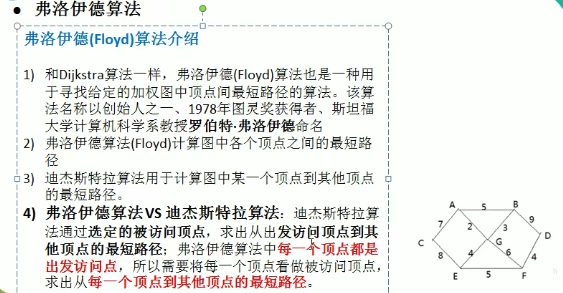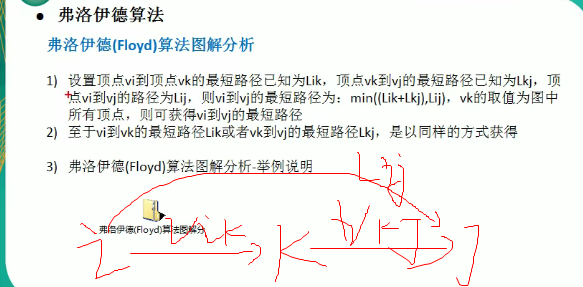## （1）先初始化两个需要的数组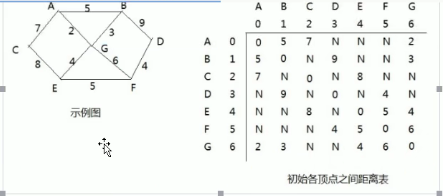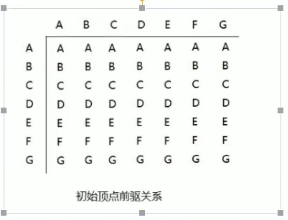## （2）找过度节点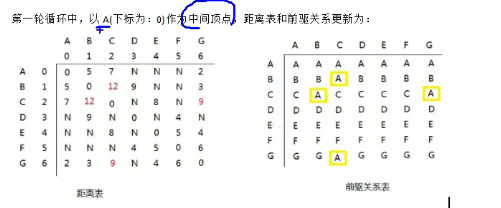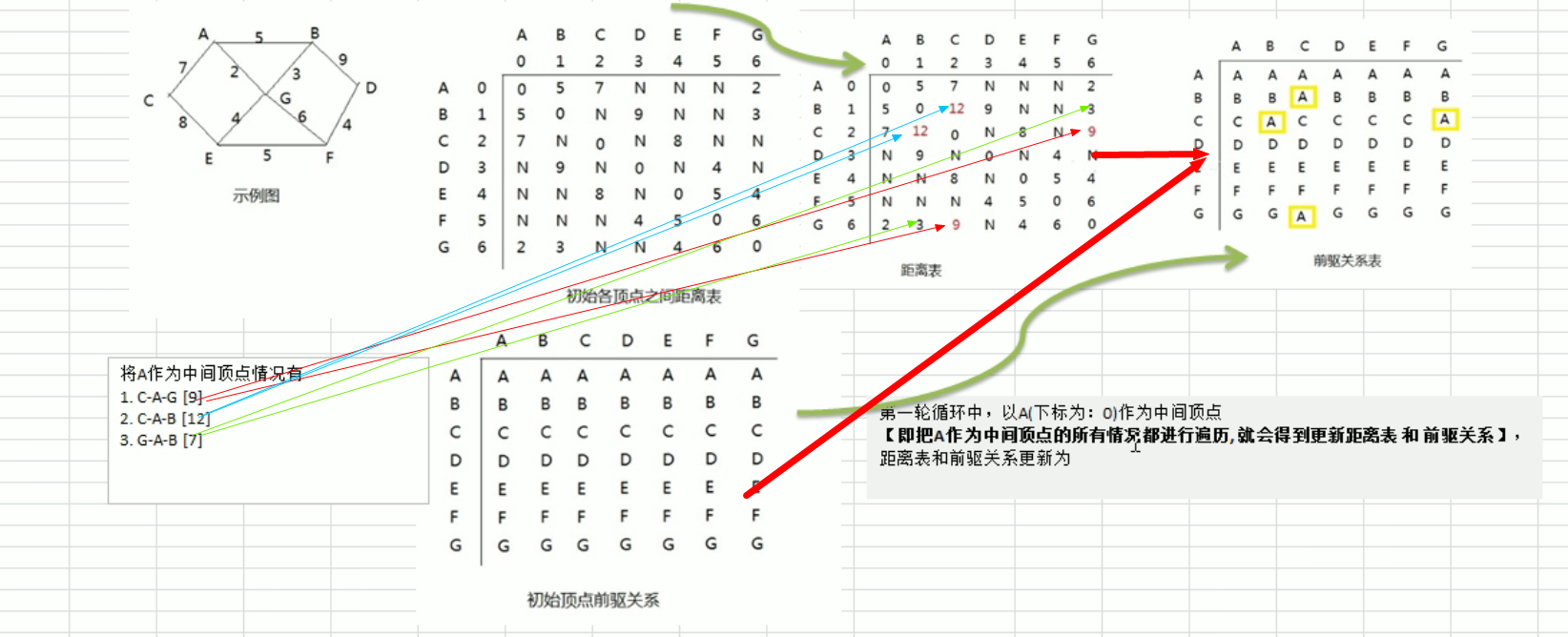## （3）总体思路

首先知道了什么是过度节点后。接下来看一下佛洛依德的算法思路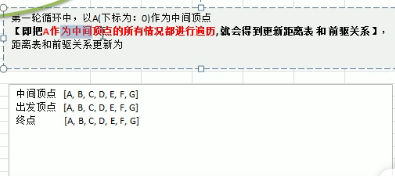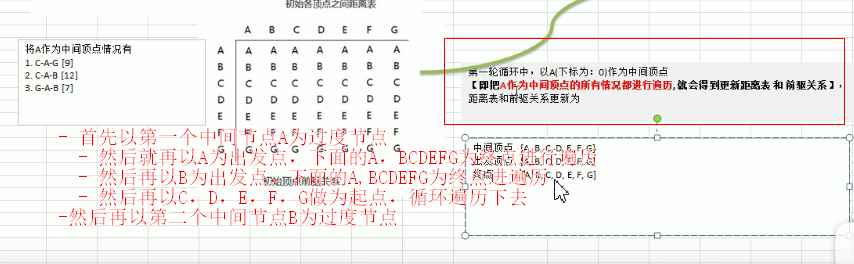• 首先以第一个中间节点A为过度节点

• 然后就再以A为出发点，
• 下面的A，BCDEFG为终点进行遍历
• 然后再以B为出发点，
• 下面的A,BCDEFG为终点进遍历
• 然后再以C，D，E，F，G做为起点，循环遍历下去
• 然后再以第二个中间节点B为过度节点，循环的往下面找

• 然后就以CDEFG为过度节点进行遍历查找

• 但是佛洛依德算法挺好，但是时间复杂度很高的，有三层for循环进行处理

最后得到的一个结果当以A为过度节点时候就得到一个最短路径，再以B作为过度节点的时候又得到一个最短路径，最后得到了每一个村庄到某一个村庄的最短距离。

## （1）题目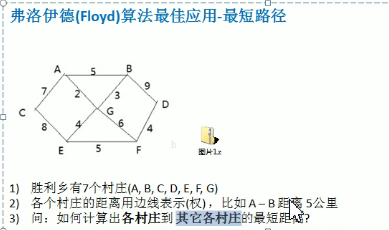• 总体的解决问题是先创建好图，并且处理好显示的效果
• 然后再进行我们佛洛依德的结果计算

## （2）图的创建

import java.util.Arrays;

public class floydDome {
public static void main(String[] args) {
//先测试图是否创建成功
char[] vertx = {'A','B','C','D','E','F','G'};
//创建领接矩阵
int[][] matrix = new int[vertx.length][vertx.length];
final int N = 65535;
matrix =new int[]{0,5,7,N,N,N,2};
matrix =new int[]{5,0,N,9,N,N,3};
matrix =new int[]{7,N,0,N,8,N,N};
matrix =new int[]{N,9,N,0,N,4,2};
matrix =new int[]{N,N,8,N,0,5,4};
matrix =new int[]{N,N,N,4,5,0,6};
matrix =new int[]{2,3,N,N,4,6,0};
//创建图对象来使用
MGraph mGraph = new MGraph(vertx.length,matrix,vertx);
mGraph.show();
}
}

class MGraph {
private char[] vertexs;//存放顶点的一个数组
private int [][] dis;//用于记录各个村庄到某个村庄的距离，最后结果也是在这里面
private int[][] pre;//保存到达目标村庄的前驱村庄,这个是动态生成的

//构造器

/**
*
* @param length 大小
* @param matrix 领接矩阵
* @param vertexs 顶点数组
*/
public MGraph(int length, int[][] matrix,char[] vertexs) {
this.vertexs = vertexs;
this.dis = matrix;
this.pre = new int[length][length];
//初始化我们的pre的前驱节点，存放的是前驱节点的下标，并不是存放的A或B什么的
for (int i = 0; i < length; i++) {
Arrays.fill(pre[i],i);
}
}

//显示dis和pre的方法
public void show() {
for (int i = 0; i < dis.length; i++) {
//显示pre数组
for (int j = 0; j < dis.length; j++) {
System.out.print(vertexs[pre[i][j]] + " ");
}
System.out.println();
//输出dis数组
for (int j = 0; j < dis.length; j++) {
System.out.print("{" +vertexs[i] + "到" + vertexs[j] + "的最短路径" + dis[i][j] + "}");
}
System.out.println();
}
}
}


## （3）佛洛依德算法

在我们的图类里面添加如下方法

//佛洛依德算法的实现
public void floyd() {
int len = 0;//变量用于记录距离

//对中间顶点的遍历，k就是中间顶点的下标也就是从A到G
for (int k = 0; k < dis.length; k++) {
//表示从i顶点开始出发，也就是从A到G
for (int i = 0; i < dis.length; i++) {
//表示从i顶点出发，要到的j节点
for (int j = 0; j < dis.length; j++) {
len = dis[i][k] + dis[k][j];//相当于求出了从i出发经过k中间顶点，到j节点的距离
if (len < dis[i][j]) {
//入过小于从i到k到j的距离小于直接从i到k的直连距离，那就更新
dis[i][j] = len;//就更新一波
//然后再更新一下前驱节点
pre[i][j] = pre[k][j];//更新前驱节点
}
}
}
}
}

展开全文• minDistanceInGraph 最短路径的两个算法：迪杰斯特拉算法和弗洛伊德算法Java
• 今天学习了这两种算法，都是用来求最小路径的算法，但是迪杰斯特拉算法只能从某个特定点到所有点的最短路径，而佛洛依德算法可以查出任意点到任意点的最小路径。 迪杰斯特拉： package dijkstra; import java....

今天学习了这两种算法，都是用来求最小路径的算法，但是迪杰斯特拉算法只能从某个特定点到所有点的最短路径，而佛洛依德算法可以查出任意点到任意点的最小路径。

# 迪杰斯特拉：

package dijkstra;

import java.util.Scanner;
import java.util.Stack;

/*
* 算法思路：通过从特定起点查找，一直查找到所有点到这个点的最小路径。
* 算法特点：因为要预防两点间的距离可能不是直接距离最短，所以处理要有些特别
* 算法处理思路：首先通过一个大的循环嵌套循环count（点）-1次，这么做是为了查找除了自身的所有点到起点的权值。
*              再通过一个for循环查找此时可达路径离起点到最近的点。把查出来点的设为已查找过。
*              接下来再通过这个最短权值的点进行for循环操作，这个for循环作用是如果经过新增v
*              顶点到达的定点比当前已知路径都短，我们更新
*              这个路径权值，并设置到这个点的前驱节点是v，让我们查询时能查出路径
*每个点都会走一次
* */
public class Dijkstra {
public static void main(String[] args) {
DijkstraArithmetic dijkstraArithmetic=new DijkstraArithmetic();
dijkstraArithmetic.dijkstra();
dijkstraArithmetic.find();

}
}

class DijkstraArithmetic{
//第一步：先准备一个图：
final int max = 65535;
int[][] graph = new int[][]{
{0, 1, 5, max, max, max, max, max, max},
{1, 0, 3, 7, 5, max, max, max, max},
{5, 3, 0, max, 1, 7, max, max, max},
{max, 7, max, 0, 2, max, 3, max, max},
{max, 5, 1, 2, 0, 3, 6, 9, max},
{max, max, 7, max, 3, 0, max, 5, max},
{max, max, max, 3, 6, max, 0, 2, 7},
{max, max, max, max, 9, 5, 2, 0, 4},
{max, max, max, max, max, max, 7, 4, 0}};
int[] D = new int; //建立一个存储起点vo到各点的权值的数组
int[] P = new int;//建立一个起点到这个点的最短路径的前驱结点
int[] fin = new int;//建立一个确认每个点是否已经被算出最短路径，以便确认它不需要被再计算,被计算过就被记为1，为就按记为0
public void dijkstra() {
/**
*      算法第一步：初始化工作
*/
int i, j, k=0;

//初始化这三个数组，
for (i = 0; i < 9; i++) {
D[i] = graph[i];//初始化权值
P[i] = 0;//初始化前驱节点,试试自己的猜想(不行，如果这样的话，后面的！=0判断会出错误，而且能联通的都不为零）
fin[i] = 0;
}
fin = 1;//不用计算起点
/*
* 第二步，通过现可达点查找最小的权值
* */
for (i = 1; i < 9; i++) {//遍历所有点的个数（除了v0）
int min = 65535;
for (j = 1; j < 9; j++) {//寻找v0最近的顶点

if (fin[j] != 1 && D[j] < min) {//确认要加入的点没有被加入过，并且某点可达且找出最小值
min = D[j];//把目前查到的最小值赋给min
k = j;//记录最小值的下标
}
}
fin[k] = 1;
for (j = 0; j < 9; j++) {//新更新了点，所以有了新的可达路径，更新这些新路径
if (fin[j] != 1 && D[j]>min +graph[k][j]){//更新那些和vo没链接但是和新的点有连接的点或者经过新的点有更近路径的点
D[j]=min+graph[k][j];//更新D[]数组的最小距离
P[j]=k;//设置修正权值的点的前驱结点
}
}
}
}
public  void find(){//输出最近距离节点
int i;
System.out.println("请输入你想要到达的结点");
Scanner sc=new Scanner(System.in);
int pointnum=sc.nextInt();
i=pointnum;
//        while(P[i]!=0){//循环输出每个点的前驱
//           System.out.print(P[i]+"<-");
//           i=P[i];
//        }
//        System.out.println("0");//输出最后一个是零点
//输出时存的是某个节点的前驱结点

//用栈输出
StackMySelf stackMySelf=new StackMySelf(15);

while(P[i]!=0){
System.out.println(P[i]);
stackMySelf.push(P[i]);//让每个元素入栈
i=P[i];
}
stackMySelf.push(0);//把起点放进去
while(!stackMySelf.empty()){
System.out.print(stackMySelf.pop());
if(!stackMySelf.empty()){
System.out.print("->");//最后一个点后面不会输出->
}
}
}
}

class StackMySelf {//自定义一个栈
private Object[] data =null;// 先自定义数组来当作栈的容器
private int maxSize;//定义栈的最大存储空间
private int top=-1;//定义栈的栈顶指针
StackMySelf(){ //构造函数来确定栈的大小,默认是10
this(10);
}
StackMySelf(int init) {//构造函数来确定栈的大小
if (init >= 0) {//如果栈的容量大于等于1
maxSize = init;//确定最大长度
data = new Object[init];//实例化数组
top = -1;//确认栈顶指针
} else {
throw new RuntimeException("初始化大小不能小于0" + init);
}
}

public boolean empty(){//判空
}
public Object pop() {//出栈
if (top == -1) { //先判断栈不为空
throw new RuntimeException("栈为空");
} else {
return data[top--];
}
}
public boolean push(Object e){//入栈
if (top == maxSize - 1) {
throw new RuntimeException("栈已满");
} else {
data[++top]=e;//赋值
return  true;
}
}
public Object peek(){//查看栈顶元素但不移除
if (top == maxSize - 1) {
throw new RuntimeException("栈已满");
} else {
return  data[top];
}
}

public int search(Object e){//返回对象在堆栈中的位置，以1为基数
int i=top;//保留top的原本值
while(top!=-1){//循环一直找到栈底
if(peek()!=e){
top--;
}else {
break;
}
}
int result=top+1;//以1开始，所以加个1
top=i;//恢复top的值
return result;
}

}/*
* 算法思路：通过从特定起点查找，一直查找到所有点到这个点的最小路径。
* 算法特点：因为要预防两点间的距离可能不是直接距离最短，所以处理要有些特别
* 算法处理思路：首先通过一个大的循环嵌套循环count（点）-1次，这么做是为了查找除了自身的所有点到起点的权值。
*              再通过一个for循环查找此时可达路径离起点最近的点。把查出来点的设为已查找过。
*              接下来再通过这个最短权值的点进行for循环操作，这个for循环作用是如果经过新增v顶点到达的定点比当前已知路径都短，我们更新
*              这个路径权值，并设置到这个点的前驱节点是v，让我们查询时能查出路径
*每个点都会走一次
* */
public class Dijkstra {
public static void main(String[] args) {
DijkstraArithmetic dijkstraArithmetic=new DijkstraArithmetic();
dijkstraArithmetic.dijkstra();
dijkstraArithmetic.find();

}
}

class DijkstraArithmetic{
//第一步：先准备一个图：
final int max = 65535;
int[][] graph = new int[][]{
{0, 1, 5, max, max, max, max, max, max},
{1, 0, 3, 7, 5, max, max, max, max},
{5, 3, 0, max, 1, 7, max, max, max},
{max, 7, max, 0, 2, max, 3, max, max},
{max, 5, 1, 2, 0, 3, 6, 9, max},
{max, max, 7, max, 3, 0, max, 5, max},
{max, max, max, 3, 6, max, 0, 2, 7},
{max, max, max, max, 9, 5, 2, 0, 4},
{max, max, max, max, max, max, 7, 4, 0}};
int[] D = new int; //建立一个存储起点vo到各点的权值的数组
int[] P = new int;//建立一个起点到这个点的最短路径的前驱结点
int[] fin = new int;//建立一个确认每个点是否已经被算出最短路径，以便确认它不需要被再计算,被计算过就被记为1，为就按记为0
public void dijkstra() {
/**
*      算法第一步：初始化工作
*/
int i, j, k=0;

//初始化这三个数组，
for (i = 0; i < 9; i++) {
D[i] = graph[i];//初始化权值
P[i] = 0;//初始化前驱节点,试试自己的猜想(不行，如果这样的话，后面的！=0判断会出错误，而且能联通的都不为零）
fin[i] = 0;
}
fin = 1;//不用计算起点
/*
* 第二步，通过现可达点查找最小的权值
* */
for (i = 1; i < 9; i++) {//遍历所有点的个数（除了v0）
int min = 65535;
for (j = 1; j < 9; j++) {//寻找v0最近的顶点

if (fin[j] != 1 && D[j] < min) {//确认要加入的点没有被加入过，并且某点可达且找出最小值
min = D[j];//把目前查到的最小值赋给min
k = j;//记录最小值的下标
}
}
fin[k] = 1;
for (j = 0; j < 9; j++) {//新更新了点，所以有了新的可达路径，更新这些新路径
if (fin[j] != 1 && D[j]>min +graph[k][j]){//更新那些和vo没链接但是和新的点有连接的点或者经过新的点有更近路径的点
D[j]=min+graph[k][j];//更新D[]数组的最小距离
P[j]=k;//设置修正权值的点的前驱结点
}
}
}
}
public  void find(){//输出最近距离节点
int i;
System.out.println("请输入你想要到达的结点");
Scanner sc=new Scanner(System.in);
int pointnum=sc.nextInt();
i=pointnum;
//        while(P[i]!=0){//循环输出每个点的前驱
//           System.out.print(P[i]+"<-");
//           i=P[i];
//        }
//        System.out.println("0");//输出最后一个是零点
//输出时存的是某个节点的前驱结点

//用栈输出
StackMySelf stackMySelf=new StackMySelf(15);

while(P[i]!=0){
System.out.println(P[i]);
stackMySelf.push(P[i]);//让每个元素入栈
i=P[i];
}
stackMySelf.push(0);//把起点放进去
while(!stackMySelf.empty()){
System.out.print(stackMySelf.pop());
if(!stackMySelf.empty()){
System.out.print("->");//最后一个点后面不会输出->
}
}
}
}

class StackMySelf {//自定义一个栈
private Object[] data =null;// 先自定义数组来当作栈的容器
private int maxSize;//定义栈的最大存储空间
private int top=-1;//定义栈的栈顶指针
StackMySelf(){ //构造函数来确定栈的大小,默认是10
this(10);
}
StackMySelf(int init) {//构造函数来确定栈的大小
if (init >= 0) {//如果栈的容量大于等于1
maxSize = init;//确定最大长度
data = new Object[init];//实例化数组
top = -1;//确认栈顶指针
} else {
throw new RuntimeException("初始化大小不能小于0" + init);
}
}

public boolean empty(){//判空
}
public Object pop() {//出栈
if (top == -1) { //先判断栈不为空
throw new RuntimeException("栈为空");
} else {
return data[top--];
}
}
public boolean push(Object e){//入栈
if (top == maxSize - 1) {
throw new RuntimeException("栈已满");
} else {
data[++top]=e;//赋值
return  true;
}
}
public Object peek(){//查看栈顶元素但不移除
if (top == maxSize - 1) {
throw new RuntimeException("栈已满");
} else {
return  data[top];
}
}

public int search(Object e){//返回对象在堆栈中的位置，以1为基数
int i=top;//保留top的原本值
while(top!=-1){//循环一直找到栈底
if(peek()!=e){
top--;
}else {
break;
}
}
int result=top+1;//以1开始，所以加个1
top=i;//恢复top的值
return result;
}

}



我在这里记下学习这个算法时一些遇到的问题：

如：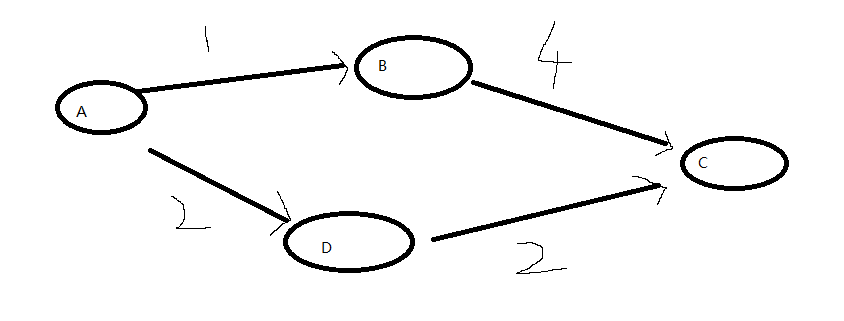如果走到了B更新了B,然后修正到c的路径，可是a从d走才是最近的，这里想了很久，最后发现，他每次循环直走一个点，并设置一个点为走过路径，也就是说修正a到c的路径时，但是并没有把c加入走过路径，这时再通过D数组里的最小值会找到d，然后修正从d走a到c的路径。如果说a先到b直接到c这种情况只可能a到d的路径比a到b到c的路径大。

# 佛洛依德算法：

package Prim;

import com.sun.corba.se.impl.orbutil.graph.Graph;
import com.sun.org.apache.xerces.internal.util.SynchronizedSymbolTable;

public class Prim {
public static void main(String[] args){
int[][] graph=new int[][]{{0,6,1,5,65535,65535},
{6,0,5,65535,3,65535},
{1,5,0,5,6,4},
{5,0,5,0,65535,2},
{65535,3,6,65535,0,6},
{65535,65535,4,2,6,0}};
MinTree minTree=new MinTree();
minTree.createMinTree(graph);
}

}

class MinTree{
public void createMinTree(int[][] graph) {

int i, j,k=0 ,m;
int[] lowcost = new int;//建立一个存储已被包入当前生成树的所有可达路径的权值
//接下来初始化第一个点
lowcost = 0;//我们要将所有访问过的节点包括设为0，以至于不会被访问到
//将第一行的数据存入lowcost中
for (i = 0; i < 6; i++) {
lowcost[i] = graph[i];//初始化到所有点的权值，访问不到的是65535，以后会有新的点来更新这个数据
}

/**
* 先选出当前可达点的权值最小的点，并加入生成树
* **/
for (i = 1; i < 6; i++) {//需要连接几个点就遍历几次
int min = 65535;//定义在这里防止min拿到了最小值，之后min无法改变
for (j = 1; j < 6; j++) {//遍历所有lowcost找到现在最小的权值
if (lowcost[j] != 0 && lowcost[j] < min) {
min = lowcost[j];//将最小权值点赋给lowcost，循环结束得到最小的
k=j;//将最小权值点的下标值存入k
}
}

lowcost[k]=0;//将这个点包入生成树
/**
* 下面的步骤：
* 作用：因为加入了新的节点，所以更新到各个点的最小权值
**/
for (m=1;m<6;m++){//将新加入生成树的点的连接点的权值全部更新至lowcost
if(graph[k][m]!=0&&graph[k][m]<lowcost[m]){//查看这个新加入点的权值是否有小于lowcost存在的权值并存入
lowcost[m]=graph[k][m];//赋值
}
}
}
}
}




# 佛洛依德算法思想就是通过三层循环，把每个点当作中继点，去连接他能连接通的所有点，直到最后一点时， 保证所有点都能有相连接路径，这里有直接连接得也有通过中继点连接的，通过另一个二维数组存储它的路径节点，用于输出。

展开全文• 佛洛依德算法佛洛依德算法佛洛依德算法佛洛依德算法佛洛依德算法佛洛依德算法佛洛依德算法佛洛依德算法佛洛依德算法佛洛依德算法佛洛依德算法佛洛依德算法佛洛依德算法佛洛依德算法佛洛依德算法佛洛依德算法佛洛依德...
• 这个方法中，其中每一个顶点到另一个顶点最多就是两步。 所以就是找到两个顶点的最近距离 package a; import java.lang.reflect.Array; import java.util.Arrays; ...public class FloydDemo { public static void ...

这个方法中，其中每一个顶点到另一个顶点最多就是两步。
所以就是找到两个顶点的最近距离

package a;

import java.lang.reflect.Array;
import java.util.Arrays;

public class FloydDemo {
public static void main(String[] args) {
char[] diots = {'A', 'B', 'C', 'D', 'E', 'F', 'G'};
int[][] edges = new int[diots.length][diots.length];
final int N = 65535;

edges=new int[]{0,5,7,N,N,N,2};//注意这个自身到自身的距离是0 ，并不是N 和地杰斯特拉的区别
edges=new int[]{5,0,N,9,N,N,3};
edges=new int[]{7,N,0,N,8,N,N};
edges=new int[]{N,9,N,0,N,4,N};
edges=new int[]{N,N,8,N,0,5,4};
edges=new int[]{N,N,N,4,5,0,6};
edges=new int[]{2,3,N,N,4,6,0};

FGraph fGraph = new FGraph(diots, edges, diots.length);
fGraph.floyd();
fGraph.show();
}
}

class FGraph{
char [] data;
int [] [] dis;
int [][] pre;

public FGraph(char[] data, int[][] dis, int len) {
this.data = data;
this.dis = dis;
pre = new int[len][len];
for (int i = 0; i < data.length; i++) {

Arrays.fill(pre[i],i);//每个顶点的前驱节点默认值是自身的下标。和地杰斯特拉不一样，地杰斯特拉默认不初始化。
}
}

public void show(){
for (int i = 0; i < data.length; i++) {
for (int j = 0; j < data.length; j++) {
System.out.print(data[pre[i][j]]+"\t");
}
System.out.println();
for (int j = 0; j < data.length; j++) {
System.out.print("("+data[i]+"-->"+data[j]+":"+dis[i][j]+"|\t");
}
System.out.println();
}

}

public void floyd(){
int len =0;//变量保存距离
for (int i = 0; i < data.length; i++) {//中间
for (int j = 0; j < data.length; j++) {//起点
for (int k = 0; k < data.length; k++) {//终点
len = dis[j][i]+dis[i][k];
if (len < dis[j][k]) {
dis[j][k] = len;
pre[j][k] = pre[i][k];//这个是更新前一个顶点。在初始化的时候pre 的每一行都是 自身。所以，这个第一次是自身。然后变成了中间的这个点。
}
}
}
}

}

}


展开全文•  另外需要注意的是：Floyd-Warshall算法不能解决带有“负权回路”（或者叫“负权环”）的图，因为带有“负权回路”的图没有最短路。例如下面这个图就不存在1号顶点到3号顶点的最短路径。因为1->2->3->1->2->3->…->...
• 1)和Dijkstra算法一 样，弗洛伊德(Floyd)算法也是一种用于寻找给定的加权图中顶点间最短路径的算法。该算法名称以创始人之一、1978年图灵 奖获得者、斯坦福大学计算机科学系教授罗伯特.弗洛伊德命名 2)弗洛伊德算法...
• 带权图的多种算法（有向图，无向图，Dijkstra算法，到每个顶点的最短距离，佛洛依德算法(Floyd)，找出每对顶点的最短路径，带权重无向图最小生成树，prim算法，Kruskal算法求最小生成树）java实现， 有注释，简单...
• 佛洛依德算法求最短路径实例 #include <iostream> #include <string.h> #include <stdlib.h> #include <stdio.h> using namespace std; //最多的地点数量 const int MAX_VALUE = 30; //...
• 怎么硕呢，最起初的动态规划，用k一直在i和j之间反颠覆找更短的，如果更短就赋值。 这不是有手就行？ //核心代码 for (k=0;k<n;k++) //计算Ak { for (i=0;i<n;i++) for (j=0;......数据结构 动态规划
• 迪杰斯特拉算法用于计算：某点v0到其他所有点的最短路径，时间复杂度为O(n^2) 初态： 　设定V为所有顶点的集合。 　设定S为已经得到的最短路径的顶点vi的集合。即S中的顶点vi，都是已经确定下来v0到vi间的最短...
• 佛洛依德算法算法作为一个经典的求最短路径的算法，思路其实很简单，就是不停地进行“松弛操作”，直到全部遍历一遍。那么什么是松弛操作呢？比如说我们的图存在一个邻接矩阵graph中，graph[v][u]为顶点v到顶点u的...c++ 数据结构与算法
• 佛洛依德算法： 利用D矩阵拿到邻接矩阵中的权值。path矩阵记录两点之间的移动中转点（初始值为起点）。 对于邻接矩阵中 i 到 j 点的权值进行比较，若加上一个中转点 k 后的权值小于原本的权值，则对D[i][j]改为较...
• 2009年甘肃省创新杯项目02应用系统设计 中 基于佛洛依德算法的各院校间最短路径问题的求解
• 1)和Dijkstra算法一 样，弗洛伊德(Floyd)算法也是一种用于寻找给定的加权图中顶点间最短路径的算法。该算法名称以创始人之一、1978年图灵 奖获得者、斯坦福大学计算机科学系教授罗伯特.弗洛伊德命名\ 2)弗洛伊德算法...python java 数据结构 c++
• 弗洛伊德(Floyd)算法介绍 和Dijkstra算法一样，弗洛伊德(Floyd)算法也是一种用于寻找给定的加权图中顶点间最短路径的算法。该算法名称以创始人之一、1978年图灵奖获得者、斯坦福大学计算机科学系教授罗伯特·...数据结构与算法
• Java实现佛洛依德算法（floyd）的完整代码 /** * 弗洛伊德(floyd)算法求图中所有点对之间的最短路径； * 其中‘-1’表示两点之间目前还没有联通的路径； * 结论：如果A点到G点之间有最短路径，那么被这条最短...图的最短路径 floyd 完整代码 Java
• package Algorithm; import java.util.Arrays; public class Floyd { public static void main(String[] args) { char [] vertex = {'A','B','C','D','E','F','G'};//顶点 final int N = 65535;...数据结构
• 1.n个点，m条边，含有负边。 1.外层循环n-1次，内层循环m次，进行松弛 3.添加check变量判断本轮是否进行松弛了，如果未进行松弛则可以提前退出循环 4.处理有向边时，注意u[i]和v[i]的顺序不要颠倒 ...
• 数据结构与算法，图，求每一对顶点间的最短路径问题，弗洛伊德算法完整实现，带注释
•matlab
• * 弗洛伊德算法思想: * Ak(i,j)意思是i点到j点经过一系列点，但是点下标最多不超过k * 情况1：如果Ak(i,j)不经过k,那么Ak(i,j)=Ak-1(i,j); * 情况2：如果Ak(i,j)经过k，那么Ak(i,j)=Ak-1(i,k)+Ak-1(k,j); * ...java
• 和Dijkstra算法一样，弗洛伊德(Floyd)算法也是一种用于寻找给定的加权图中顶点间最短路径的算法。该算法名称以创始人之一、1978年图灵奖获得者、斯坦福大学计算机科学系教授罗伯特·弗洛伊德命名。 基本思想 &...
• 处理的是在几个村庄之间建一个医院，使路径最短的问题，事实上也就是处理图中最短路径的问题，采用的是弗洛伊德算法...